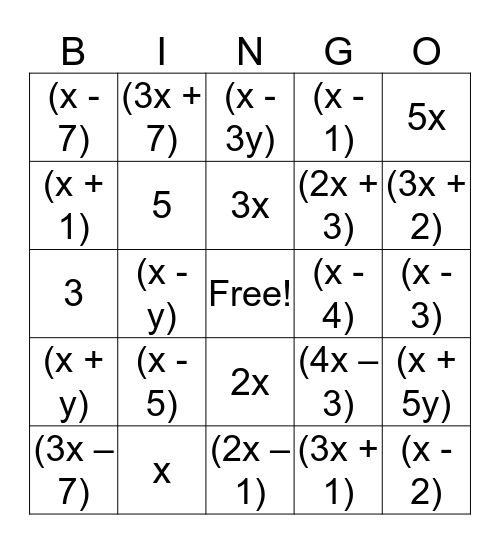# Factoring Bingo!This bingo card has a free space and 41 words: 2, 3, 4, 5, x, 2x, 3x, 4x, 5x, (x + y), (x - y), (x - 1), (x - 2), (x - 3), (x - 4), (x - 5), (x - 6), (x - 7), (x - 5y), (x - 3y), (x + 5y), (2x + 1), (2x – 1), (2x + 3), (2x – 3), (2x + 5), (3x + 1), (3x – 1), (3x + 2), (3x + 4), (3x – 4), (3x + 7), (3x – 7), (4x + 1), (4x – 3), (x + 1), (x + 2), (x + 3), (x + 5), (x + 6) and (x + 9).

## Play Online